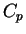Next: Updating the network weights Up: Learning algorithm for the Previous: Evaluating the cost function   Contents

## Optimising the cost function

The most difficult part in optimising the cost function for the NSSM is updating the hidden states and the weights of the MLP networks. All the hyperparameters can be handled in exactly the same way as in the CDHMM case presented in Section 6.1.2 but ignoring the additional weights caused by the HMM state probabilities.

Updating the states and the weights is carried out in two steps. First the value of the cost function is evaluated using the current estimates for all the variables. This is called forward computation because it consists of a forward pass through the MLP networks.

The second, backward computation step consists of evaluating the partial derivatives of thepart of the cost function with respect to the different parameters. This can be done by moving backward in the network, starting from the outputs and proceeding toward the inputs. The standard back-propagation calculations are done in the same way. In our case, however, all the parameters are described by their own posterior distributions, which are characterised by their means and variances. The cost function is very different from the standard back-propagation and the learning is unsupervised. This means that all the calculation formulas are different.

SubsectionsNext: Updating the network weights Up: Learning algorithm for the Previous: Evaluating the cost function   Contents
Antti Honkela 2001-05-30